# Introduction to Vibration of Multi Degree of Freedom Systems (Part - 2) Civil Engineering (CE) Notes | EduRev

## Civil Engineering (CE) : Introduction to Vibration of Multi Degree of Freedom Systems (Part - 2) Civil Engineering (CE) Notes | EduRev

The document Introduction to Vibration of Multi Degree of Freedom Systems (Part - 2) Civil Engineering (CE) Notes | EduRev is a part of the Civil Engineering (CE) Course Introduction to Dynamics and Vibrations- Notes, Videos, MCQs.
All you need of Civil Engineering (CE) at this link: Civil Engineering (CE)

5.5.5 The effects of damping

In most design calculations, we don’t worry about accounting for the effects of damping very accurately. This is partly because it’s very difficult to find formulas that model damping realistically, and even more difficult to find values for the damping parameters. Also, the mathematics required to solve damped problems is a bit messy. Old textbooks don’t cover it, because for practical purposes it is only possible to do the calculations using a computer. It is not hard to account for the effects of damping, however, and it is helpful to have a sense of what its effect will be in a real system. We’ll go through this rather briefly in this section.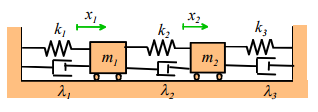Equations of motion: The figure shows a damped spring-mass system. The equations of motion for the system can easily be shown to be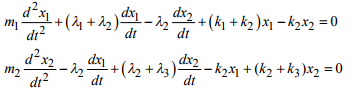To solve these equations, we have to reduce them to a system that MATLAB can handle, by re-writing them as first order equations. We follow the standard procedure to do this – define v1 = dx1 / dt and v2 = dx2 / dt as new variables, and then write the equations in matrix form as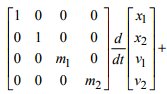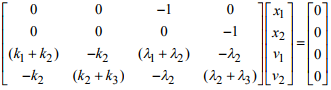(This result might not be obvious to you – if so, multiply out the vector-matrix products to see that the equations are all correct). This is a matrix equation of the form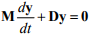where y is a vector containing the unknown velocities and positions of the mass.

Free vibration response: Suppose that at time t=0 the system has initial positions and velocities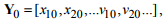and we wish to calculate the subsequent motion of the system. To do this, we must solve the equation of motion. We start by guessing that the solution has the form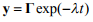(the negative sign is introduced because we expect solutions to decay with time). Here,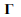is a constant vector, to be determined. Substituting this into the equation of motion gives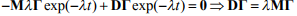This is another generalized eigenvalue problem, and can easily be solved with MATLAB. The solution is much more complicated for a damped system, however, because the possible values of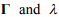that satisfy the equation are in general complex – that is to say, each λ can be expressed as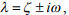where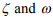are positive real numbers, and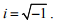This makes more sense if we recall Euler’s formula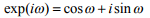(if you haven’t seen Euler’s formula, try doing a Taylor expansion of both sides of the equation – you will find they are magically equal. If you don’t know how to do a Taylor expansion, you probably stopped reading this ages ago, but if you are still hanging in there, just trust me…). So, the solution is predicting that the response may be oscillatory, as we would expect. Once all the possible vectors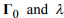have been calculated, the response of the system can be calculated as follows:

1. Construct a matrix H , in which each column is one of the possible values of(MATLAB constructs this matrix automatically)
2. Construct a diagonal matrix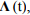which has the form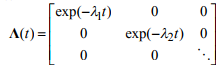where each λ is one of the solutions to the generalized eigenvalue equation.

3. Calculate a vector a (this represents the amplitudes of the various modes in the vibration response) that satisfies

Ha = Y0

4. The vibration response then follows as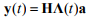All the matrices and vectors in these formulas are complex valued – but all the imaginary parts magically disappear in the final answer.

HEALTH WARNING: The formulas listed here only work if all the generalized eigenvalues λ satisfying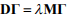are different. For some very special choices of damping, some eigenvalues may be repeated. In this case the formula won’t work. A quick and dirty fix for this is just to change the damping very slightly, and the problem disappears. Your applied math courses will hopefully show you a better fix, but we won’t worry about that here.

This all sounds a bit involved, but it actually only takes a few lines of MATLAB code to calculate the motion of any damped system. As an example, a MATLAB code that animates the motion of a damped spring-mass system shown in the figure (but with an arbitrary number of masses) can be downloaded here. You can use the code to explore the behavior of the system. In addition, you can modify the code to solve any linear free vibration problem by modifying the matrices M and D.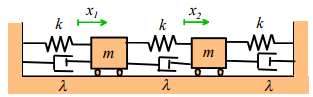Here are some animations that illustrate the behavior of the system. The animations below show vibrations of the system with initial displacements corresponding to the three mode shapes of the undamped system (calculated using the procedure in Section 5.5.2). The results are shown for k=m=1 λ =  0.05 . In each case, the graph plots the motion of the three masses – if a color doesn’t show up, it means one of the other masses has the exact same displacement.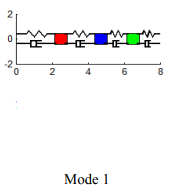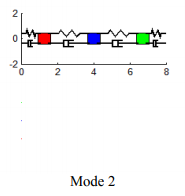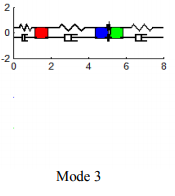Notice that

1. For each mode, the displacement history of any mass looks very similar to the behavior of a damped, 1DOF system.

2. The amplitude of the high frequency modes die out much faster than the low frequency mode. This explains why it is so helpful to understand the behavior of a 1DOF system. If a more complicated system is set in motion, its response initially involves contributions from all its vibration modes. Soon, however, the high frequency modes die out, and the dominant behavior is just caused by the lowest frequency mode. The animation to the right demonstrates this very nicely – here, the system was started by displacing only the first mass. The initial response is not harmonic, but after a short time the high frequency modes stop contributing, and the system behaves just like a 1DOF approximation. For design purposes, idealizing the system as a 1DOF damped spring-mass system is usually sufficient.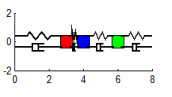Notice also that light damping has very little effect on the natural frequencies and mode shapes – so the simple undamped approximation is a good way to calculate these.

Of course, if the system is very heavily damped, then its behavior changes completely – the system no longer vibrates, and instead just moves gradually towards its equilibrium position. You can simulate this behavior for yourself using the matlab code – try running it with λ = 5 or higher. Systems of this kind are not of much practical interest.

Steady-state forced vibration response. Finally, we take a look at the effects of damping on the response of a spring-mass system to harmonic forces. The equations of motion for a damped, forced system are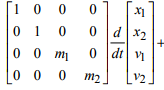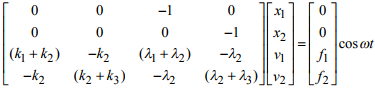This is an equation of the form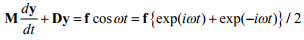where we have used Euler’s famous formula again. We can find a solution to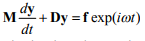by guessing that y = Y0exp(iωt), and substituting into the matrix equation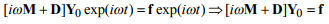This equation can be solved for Y0 . Similarly, we can solve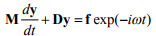by guessing that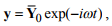which gives an equation for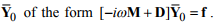You actually don’t need to solve this equation – you can simply calculate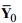by just changing the sign of all the imaginary parts of Y0 . The full solution follows asThis is the steady-state vibration response. Just as for the 1DOF system, the general solution also has a transient part, which depends on initial conditions. We know that the transient solution will die away, so we ignore it.

The solution for y(t) looks peculiar, because of the complex numbers. If we just want to plot the solution as a function of time, we don’t have to worry about the complex numbers, because they magically disappear in the final answer. In fact, if we use MATLAB to do the computations, we never even notice that the intermediate formulas involve complex numbers. If we do plot the solution, it is obvious that each mass vibrates harmonically, at the same frequency as the force (this is obvious from the formula too). It’s not worth plotting the function – we are really only interested in the amplitude of vibration of each mass. This can be calculated as follows

1.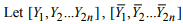denote the components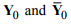2. The vibration of the jth mass then has the form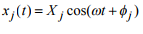where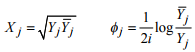are the amplitude and phase of the harmonic vibration of the mass. If you know a lot about complex numbers you could try to derive these formulas for yourself. If not, just trust me – your math classes should cover this kind of thing. MATLAB can handle all these computations effortlessly. As an example, here is a simple MATLAB script that will calculate the steady-state amplitude of vibration and phase of each degree of freedom of a forced n degree of freedom system, given the force vector f, and the matrices M and D that describe the system.

function [amp,phase] = damped_forced_vibration(D,M,f,omega)
% Function to calculate steady state amplitude of
% a forced linear system.
% D is 2nx2n the stiffness/damping matrix
% M is the 2nx2n mass matrix
% f is the 2n dimensional force vector
% omega is the forcing frequency, in radians/sec.
% The function computes a vector ‘amp’, giving the amplitude of
% each degree of freedom, and a second vector ‘phase’,
% which gives the phase of each degree of freedom
%
Y0 = (D+M*i*omega)\f; % The i here is sqrt(-1)
% We dont need to calculate Y0bar - we can just change the sign of
% the imaginary part of Y0 using the 'conj' command
for j =1:length(f)/2
amp(j) = sqrt(Y0(j)*conj(Y0(j)));
phase(j) = log(conj(Y0(j))/Y0(j))/(2*i);
end

end

Again, the script is very simple.

Here is a graph showing the predicted vibration amplitude of each mass in the system shown. Note that only mass 1 is subjected to a force.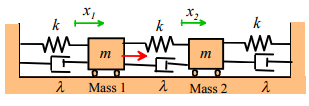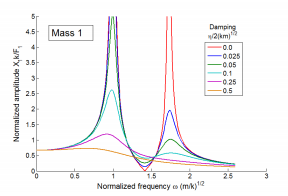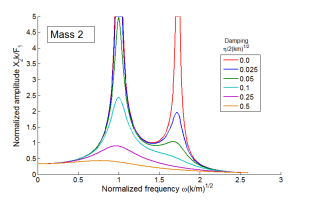The important conclusions to be drawn from these results are:

1. We observe two resonances, at frequencies very close to the undamped natural frequencies of the system.

2. For light damping, the undamped model predicts the vibration amplitude quite accurately, except very close to the resonance itself (where the undamped model has an infinite vibration amplitude)

3. In a damped system, the amplitude of the lowest frequency resonance is generally much greater than higher frequency modes. For this reason, it is often sufficient to consider only the lowest frequency mode in design calculations. This means we can idealize the system as just a single DOF system, and think of it as a simple spring-mass system as described in the early part of this chapter. The relative vibration amplitudes of the various resonances do depend to some extent on the nature of the force – it is possible to choose a set of forces that will excite only a high frequency mode, in which case the amplitude of this special excited mode will exceed all the others. But for most forcing, the lowest frequency one is the one that matters.

4. The ‘anti-resonance’ behavior shown by the forced mass disappears if the damping is too high.

Offer running on EduRev: Apply code STAYHOME200 to get INR 200 off on our premium plan EduRev Infinity!

## Introduction to Dynamics and Vibrations- Notes, Videos, MCQs

20 videos|53 docs

,

,

,

,

,

,

,

,

,

,

,

,

,

,

,

,

,

,

,

,

,

;